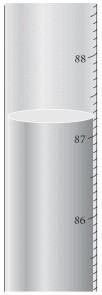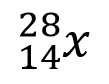# Chemistry: Final Flashcards

Set Details Share
created 7 years ago by sydneyfrench13
1,495 views
updated 7 years ago by sydneyfrench13
Subjects:
science, chemistry, physics, atomic & molecular
Page to share:
Embed this setcancel
COPY
code changes based on your size selection
Size:
X

1

Give the composition of water

A. two hydrogen atoms and two oxygen atoms

B. one hydrogen atom and one oxygen atom

C. two hydrogen atoms and one oxygen atom

D. one hydrogen atom and two oxygen atoms

C

2

Dalton's Atomic Theory states

A. That elements may have several isotopes

B. that matter is composed of small indestructible particles

C. A hypothesis is speculation that is difficult to test

D. An observation explains why nature does something

E. A scientific law summarizes a series of related observations

B

3

Which of the following statements is true

A. a scientific law is fact

B. once a theory is constructed, it is considered fact

C. a hypothesis is speculation that is difficult to test

D. an observation explains why nature does something

E. a scientific law summarizes a series of related observations

E

4

A substance that can't be chemically broken down into simpler substances is

A. a homogeneous mixture

B. an element

C. a heterogeneous mixture

D. a compound

E. an electron

B

5

A chemical change

A. occurs when methane gas is burned

B. occurs when paper is shredded

C. occurs when water is vaporized

D. occurs when salt is dissolved in water

E. occurs when powdered lemonade is stirred into water

A

6

All of the following are SI base units of measurement, except

A. meter

B. gram

C. second

D. Kelvin

E. mole

B

7

A student performs an experiment to determine the density of a sugar solution. She obtains the following results: 1.11 g/mL, 1.81 g/mL, 1.95 g/mL, 1.75 g/mL. If the actual value for the density of the sugar solution is 1.75 g/mL, which statement below best describes her results?

A. her results are precise, but not accurate

B. her results are accurate, but not precise

C. her results are broth precise and accurate

D.her results are neither precise and accurate

E. it isn't possible to determine the information given

D

8Read the temperature with the correct number of significant figures

A. 87 oC

B. 87.2 oC

C. 87.20 oC

D. 87.200 oC

E. 87.2000 oC

C

9

What answer should be reported, with the correct number of significant figures, for the following calculation (249.362 + 41) / 63.498

A. 4.6

B. 4.57

C. 4.573

D. 4.5728

E. 4.57277

B

10

If the walls in a room are 955 square feet in area, and a gallon of paint covers 15 square yards, how many gallons of paint are needed for the room (3ft = 1 yd)

A. 47 gallons

B. 21 gallons

C. 7.1 gallons

D. 24 gallons

E. 2.3 gallons

C

11

When two elements form two different compounds, the masses of element B that combine with 1 g of element A can be expressed as a ratio of small whole numbers. Which law does this refer to?

A. Law of Definite Proportions

B. Law of the Conservation of Mass

C. Law of Modern Atomic Theory

D. Law of Multiple Proportions

E. First Law of Thermodynamics

D

12What does "X represent in the following symbol

A. silicon

B. sulfur

C. zinc

D. ruthenium

E. nickel

A

13

Which of the following statements about isotopes is true

A. isotopes of the same element differ only in the number of electrons they contain

B. an isotope of an atom with a larger number of neutrons is larger than an isotopes of the same atom that contains fewer neutrons

C. isotopes of the same element have the same mass

D. isotopes of the same element don't usually have the same properites

E. some elements have 3 or more naturally occurring isotopes

E

14

What species is represented by the following information

P+= 17 no = 18 e-= 18

A. Cl

B.Cl-

C. Ar

D. Ar+

E. Kr

B

15

Predict the charge that a calcium ion would have

A. 6 -

B. 2 -

C. 3 +

D. 2 +

E. 1 +

D

16

Which of the following statements is false

A. Halogens are very reactive elements

B. The alkali metals are fairly unreactive

C. Sulfur is a main group element

D. noble gases do not usually form ions

E. An is a transition metal

B

17

Calculate the atomic mass of gallium if gallium has 2 naturally occurring isotopes with the following masses and natural abundances:

Ga-69 68.9256 amu 60.11%

Ga-71 70.9247 amu 39.89%

A. 69.72

B. 69.93

C. 70.00

D. 69.80

E. 70.68

A

18

how many moles of Cs are contained in 595 kg of Cs

A. 2.23 x 102 moles Cs

B. 4.48 x 103 moles Cs

C. 7.91 x 104 moles Cs

D. 1.26 x 103 moles Cs

E. 5.39 x 102 moles Cs

B

19

Calculate the mass (in kg) of 4.87 x 1025 atoms of Zn

A. 5.29 kg

B. 1.89 kg

C. 8.09 kg

D. 1.24 kg

E. 1.09 kg

A

20

How many xenon atoms are contained in 2.36 moles of Xenon

A. 3.92 x 1024

B. 2.55 x 1023

C. 1.42 x 1024

D. 7.91 x 1025

E. 1.87 x 1026

C